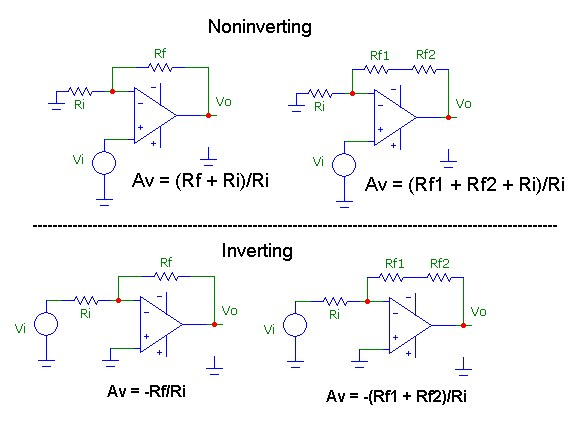## MatchGain: Find best feedback resistor match for opamp amplifiers

May 21, 2008

MatchGain is a simple console C# application. The required closed loop gain for the basic inverting and/or noninverting opamp circuit is specified, along with an optional accuracy parameter (default is 0.1% match) and matchgain returns all feedback resistor matches (with a minimum resistor value of 100 ohm) within this accuracy using the standard set of resistor values. The match accuracy should be considered within the context of the resistor tolerances, typically 5% .```Usage:
matchgain.exe <gain> [n | i | ni | n1| n2 | i1 | i2 | ni1 | ni2]  [% accuracy]

<gain> is voltage gain number Av,  or dB gain string:  20*Lgt(Av)
[% accuracy]    default is 0.1%
"n" = noninverting;  "i" = inverting;  "ni" = noninverting & inverting
"n1" noninverting with one Rf resistor
"n2" noninverting with two Rf resistors in series
"i1" inverting with one Rf resistor
"i2" inverting with two Rf resistors in series
"ni1" noninverting & inverting  with one Rf resistor
"ni2" noninverting & inverting with two Rf resistors in series

------ Examples: ------
matchgain  30
Calculates one and two resistor Rf, Ri matches for both noninverting/inverting
amplifiers with gain of 30 using default accuracy 0.1%

matchgain  20db  n1   2
Calculates Rf, Ri matches for noninverting amplifier with 20dB gain within 2%

matchgain  50  ni   0.05
Calculates one and two resistor Rf, Ri matches for both noninverting/inverting
amplifiers with gain of 50 within 0.05%
```
Downloads
matchgain.exe (requires .NET Runtime)

Is .NET Installed?
To check if the .NET runtime is installed:
• Execute the application from a command prompt: matchgain.exe without any arguments; help information returned indicates that .NET is installed